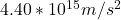## The electric field between the plates of the cathode ray tube of an older television set can be as high as 2.5×104 N/C. Determine the force

Question

The electric field between the plates of the cathode ray tube of an older television set can be as high as 2.5×104 N/C. Determine the force (use dimensional analysis!) and resulting acceleration of an electron?

in progress 0
2 months 2021-07-30T01:17:59+00:00 1 Answers 5 views 0

(a)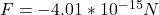(b)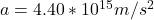Explanation:

Parameter given:

Electric field, E =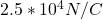(a) Electric force is given (in terms of electric field) as a product of electric charge and electric field.

Mathematically: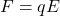Electric charge, q, of an electron =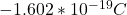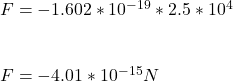(b) This electrostatic force causes the electron to accelerate with an equivalent force:

F = -ma

where m = mass of an electron

a = acceleration of electron

(Note: the force is negative cos the direction of the force is opposite the direction of the electron)

Therefore: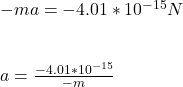Mass, m, of an electron =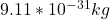=>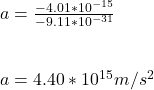The acceleration of the electron is